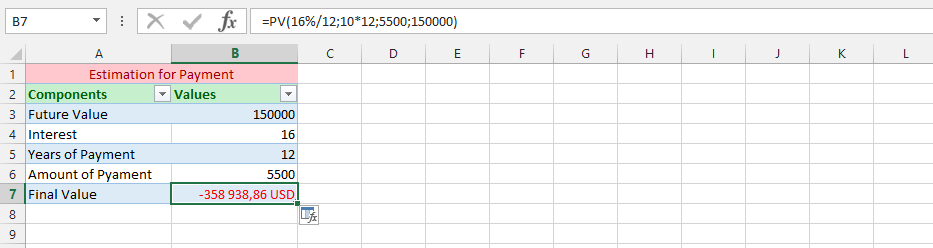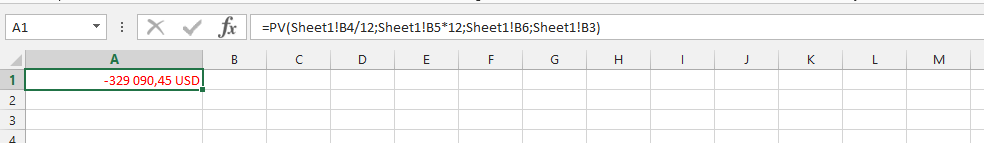#### How to Calculate Pv in Excel

The PV function in Microsoft Excel has a primary option, which is to calculate a current value of an investment that is being made. The function execute these things based on multiple payments that will be made in the future. Also you will get to know what is pv.

## Present Value function Parameters

The parameter that the Microsoft Excel includes are rate, followed by nper, then pmt, fv (future value), and type.

Rate: This is the rate of interest for each period, and a required part of the PV formula.

Nper: This is a quantity of periods for either annuities or investment that has been made, and a required component to the PV formula.

Pmt: This is a specific payment for each period, and surprisingly it is optional. If the PMT has been omitted, then it is essential for fv argument to be supplied to the PV’s formula.

[Fv]: This is specifically for informing the formula about future value of annual, which would be at end of nper payments. If this has been omitted, then it would standardly be set to a value of 0.

[Type]: This is the argument that can only be given one argument, which are either 0 or 1. 0 means that payment would be made by ending of the period, while 1 means that it is at a beginning of the period. If this argument has been omitted, then it would standardly be set to a value of 0.

## PV function examples

### Simple PV Formula

The business has set a new goal, and would like to know the present value of a business deal that we are about to make. We ask ourselves, what is the current value of it, so we could decide if the business is beneficial or not. This is where the PV function would be very useful for the successfulness of acknowledging the present value.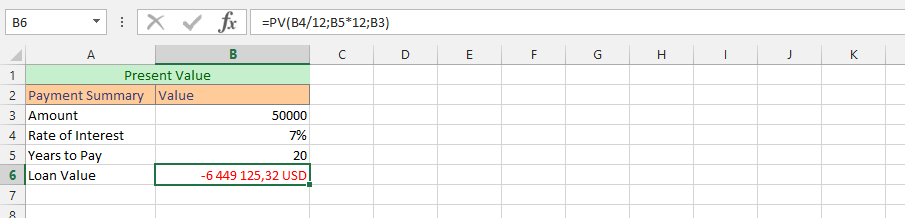### Complete PV Formula

This is an example where we will focus on finding the current value of the business. This is where the PV formula would be extremely useful. Let’s use the full formula. This example will provide us with the information we need to successfully confirm the present value of our business.

We also need to estimate the future value. This is an example that comprehensively shows us the possibilities of the pv function.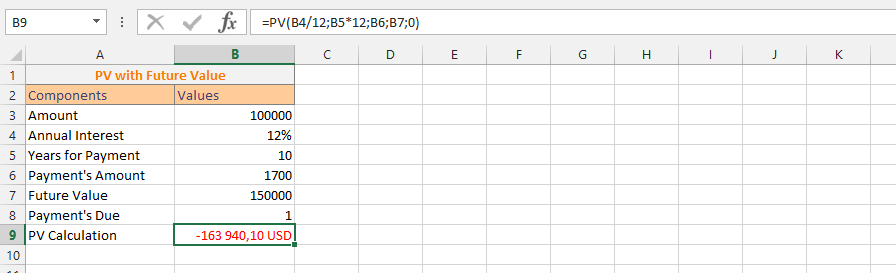### Present Value with Mathematical Symbol

We want to make a decision whether to carry out a transaction. We evaluate the value of the present value using the data we have.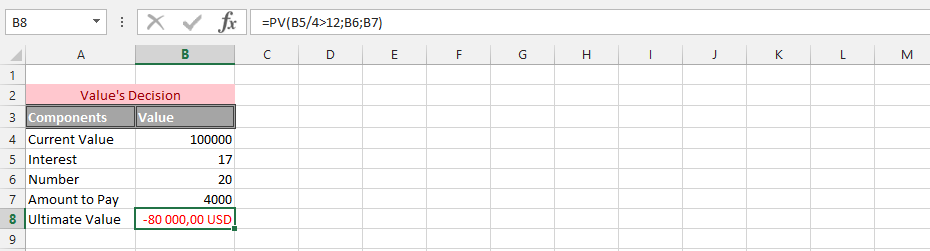### Evaluating Present Value with Additional Percentage

In this example, we have decided that we want to add a percentage to the current value. This is where the PV formula comes in extremely handy. The formula below shows the percentage that has been added to the formula.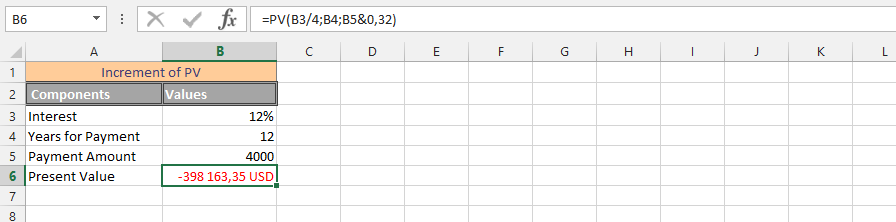### Using PV & AND Function Simultaneously

In this example, we want to find the answers we seem to have missed about the current value of the payment. There are estimates of the present value and we need to know if this estimate is correct or not. This example uses both the PV and AND functions. We also estimated the future value to be 150,000, so we need to know the current value based on that.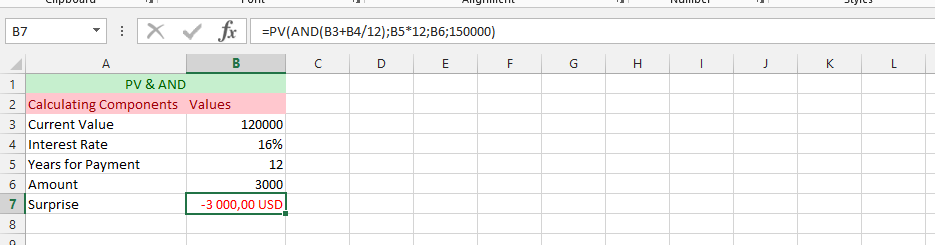### Doubled Rate with Present Value

The present value should be assessed as we expect interest rates to double in the near term. The example explains that this possibility could happen in about three years, so we are now adding it to the present value.

We believe that for the success of these calculations, PV would be an excellent strategy for confirming this information. It is very easy to confirm this information with a formula.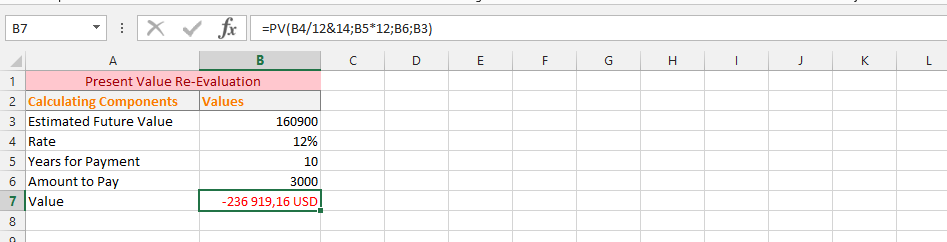### Acknowledging the PV with Text

The current value has been estimated, but in this case we used text rather than numbers. Various explanations have emerged that led us to the necessity to use the text.

The use of text is also possible for the value of PV (present value), and these possibilities have proven to be productive in the formula presented. Now we’re going to use the PV formula with text.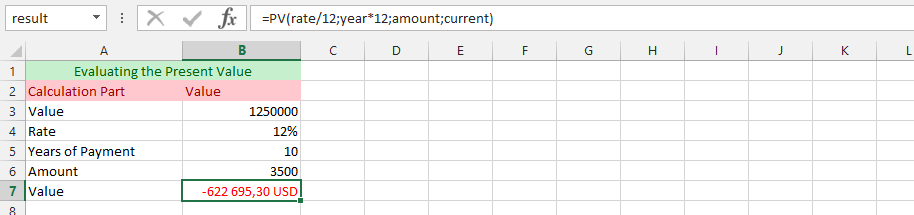### Present Value and OR Functions Simultaneously

It has been estimated that it is imperative that the present value be flexible over the next six months. We would like to divide the remaining payments into subsequent months, but the PV formula does not allow for the use of 6 months.

This makes creativity a fantastic way to solve this problem. Instead of 6 months, which he does not agree to, we use 6 years and divide it by 6 as a solid solution to the current problem.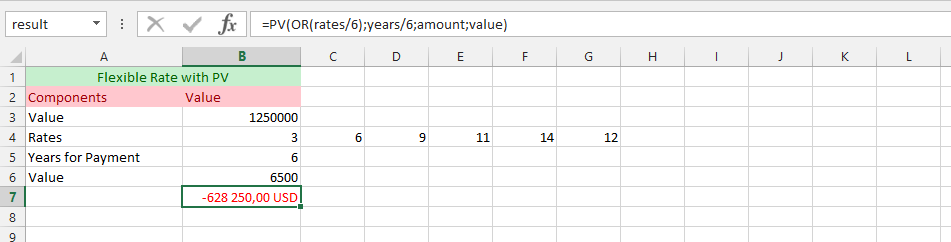### Just Numbers in Formula

The present values are being estimated with different formulas, so we are rather adding numbers than assigning the parameters with cells. This is where the example directly and sophisticatedly tackles the situation with regards to that estimations, as they have been outlaid.